Note: This version of the model includes vaccinations, based on the following data and assumptions:

• As of 3/17/2021, of the overall LA County population, 1,057,794 = 10% of the population have received 1st and 2nd doses
• As of 3/8/2021, of the population of individuals 65 and over, 815,271 = 60% have received at least their 1st dose.

We make the following assumptions to fit our model to this data:

Assumptions: General population - We model only full protection from the vaccine, i.e. 1st and 2nd doses of Moderna and Pfizer or 1 dose of J&J. - We assume 10,000 vax / day during January and February, and 20,000 vax / day from March 1 - Sys.Date(). This equals approximately 1,057,794 1st and 2nd doses by 3/17/21

Assumptions: 65+ population - We estimate that by 3/8/2021, 50% of individuals 65+ that have received at least a 1st dose have received their 2nd dose, i.e. 407,636 of the 815,271 have received both their 1st and 2nd doses by 3/8/2021. - We assume 3,500 vax / day during January and February, and 7,000 vax / day from March 1 - Sys.Date(). This equals approximately 407,636 1st and 2nd doses by 3/8/21. We estimate that the same rate continues through to Sys.Date().

Please see the last two sub-figures in the Model Fits figure for a visual of these timelines.

## Numbers infected

• New = new daily incidence
• Current = current census in compartment
• Cumulative = running total over time
• Black dots depict COVID-19 data

### Current observed and total infections (together)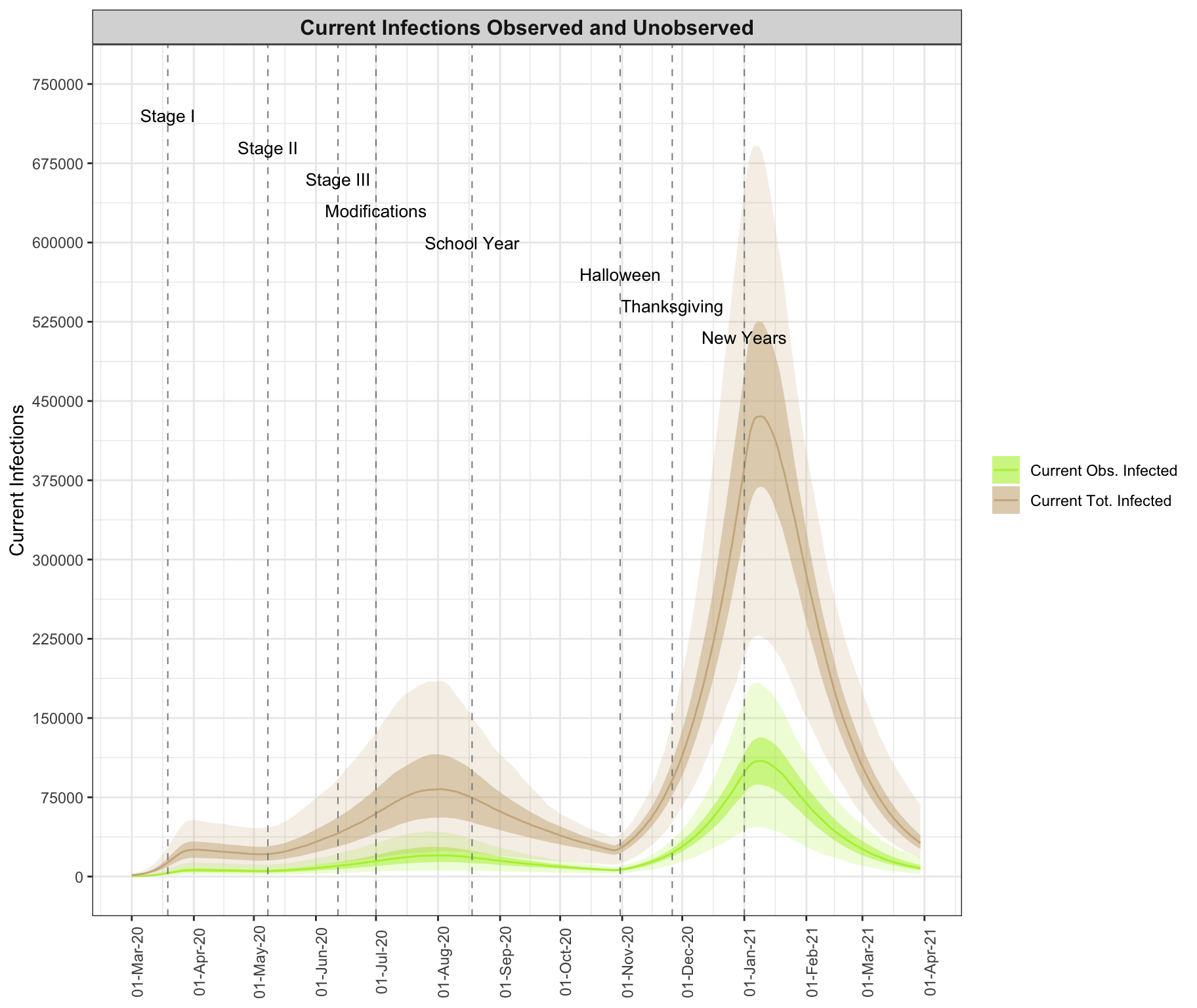{-}

## Numbers of Hospitalizations, ICU admissions, Deaths

• New = new daily incidence
• Current = current census in compartment
• Cumulative = running total over time
• Black dots depict COVID-19 data
• Dotted black line marks healthcare capacity limits

### Current hospitalizations, ventilations, and new deaths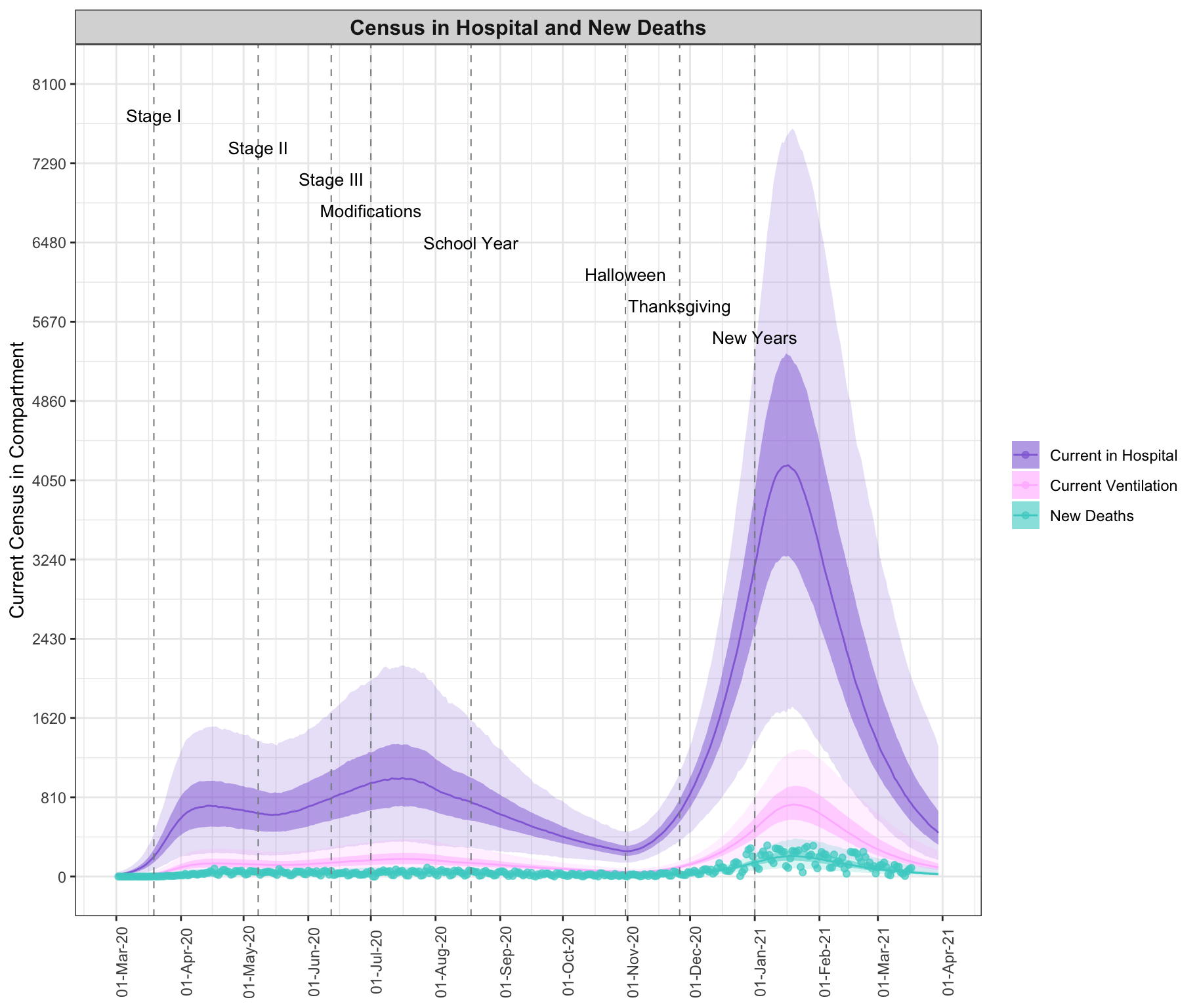## Model fits

Summarizes the epidemic model fit with COVID-19 data for LAC from March 1 through 2021-03-18 for all disease states across multiple views: New cases, representing new daily incidence; the current number in a compartment at a specific date, relevant for understanding current prevalence rates and comparing with healthcare capacity limitations; and cumulative counts until a specific date. Observed data are plotted as black dots. The figure demonstrates that good model fits are achieved in all compartments across time.

• New = new daily incidence
• Current = current census in compartment
• Cumulative = running total over time
• Black dots depict COVID-19 data
• The dashed line represents healthcare resource capacity limits

### Model fits through 2021-03-20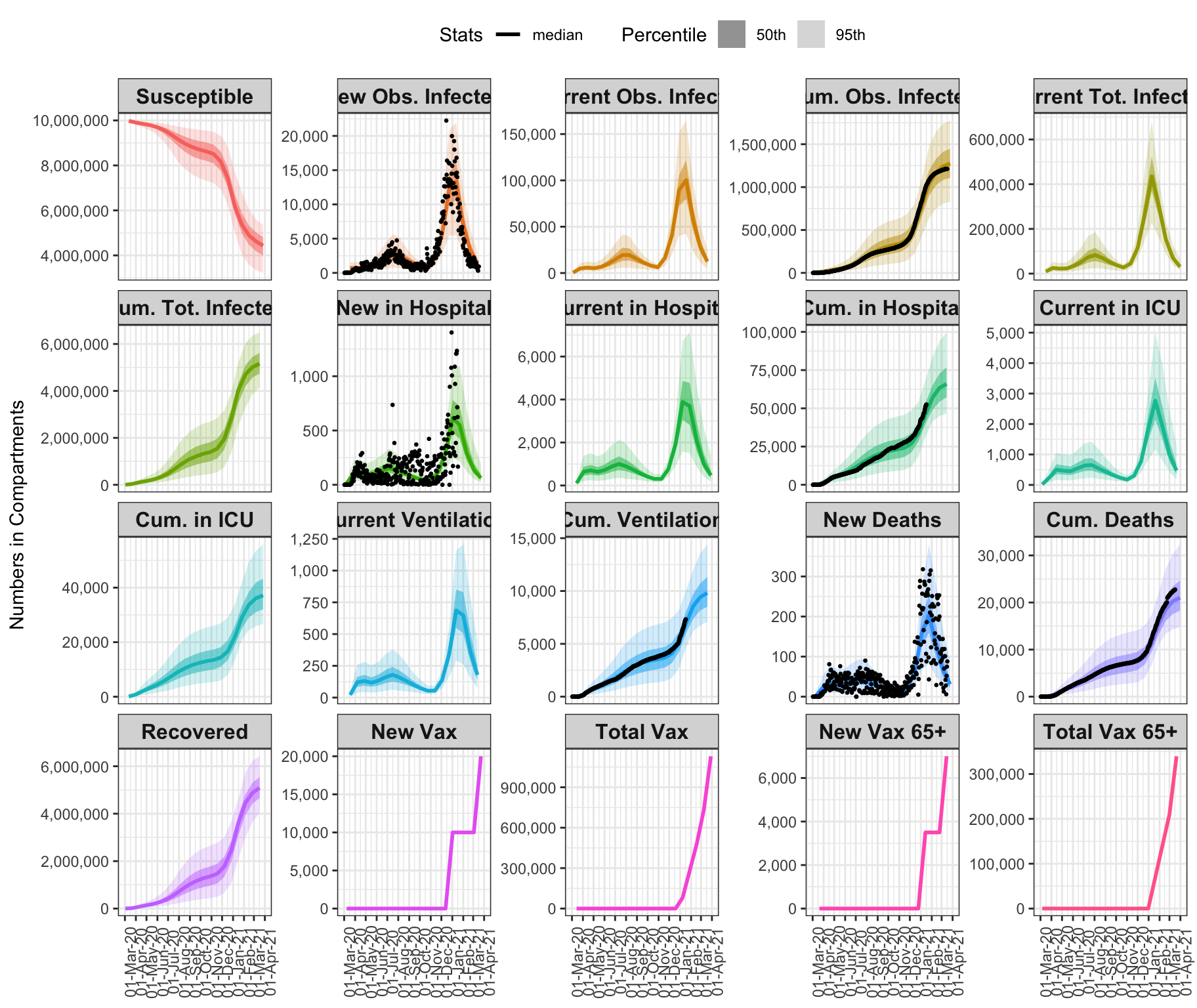### Model projections through 2021-03-30

Projections under the assumption of the infectious rate as of 2021-03-20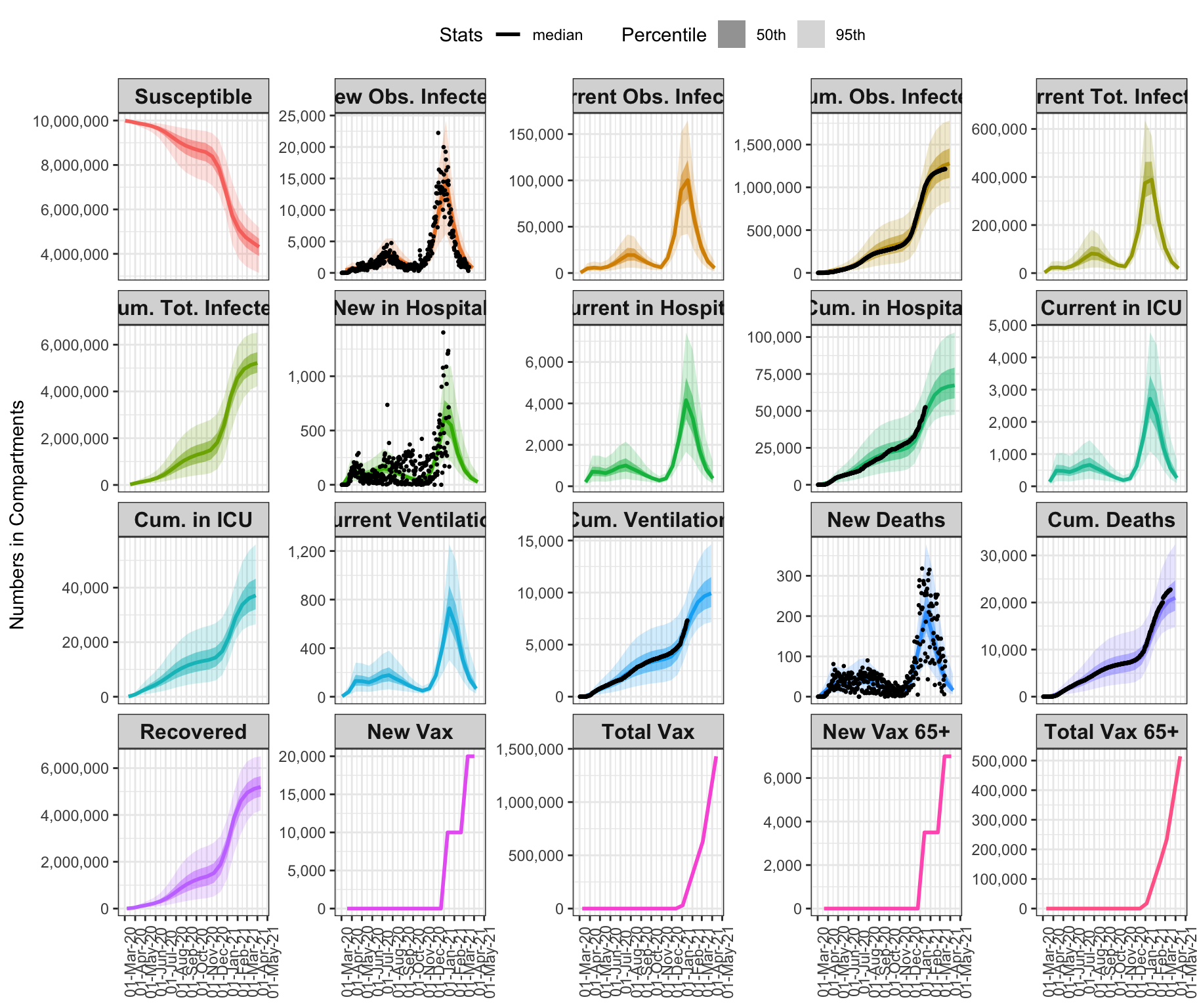{-}

## Estimated epidemic parameters

### Reproductive Number, $$R(t)$$

This plot shows the time-varying Reproductive Number R(t) but NOT its effective value. This means it does NOT account for herd immunity. What is presented here is an indication of how much transmission is happening, without accounting for herd immunity.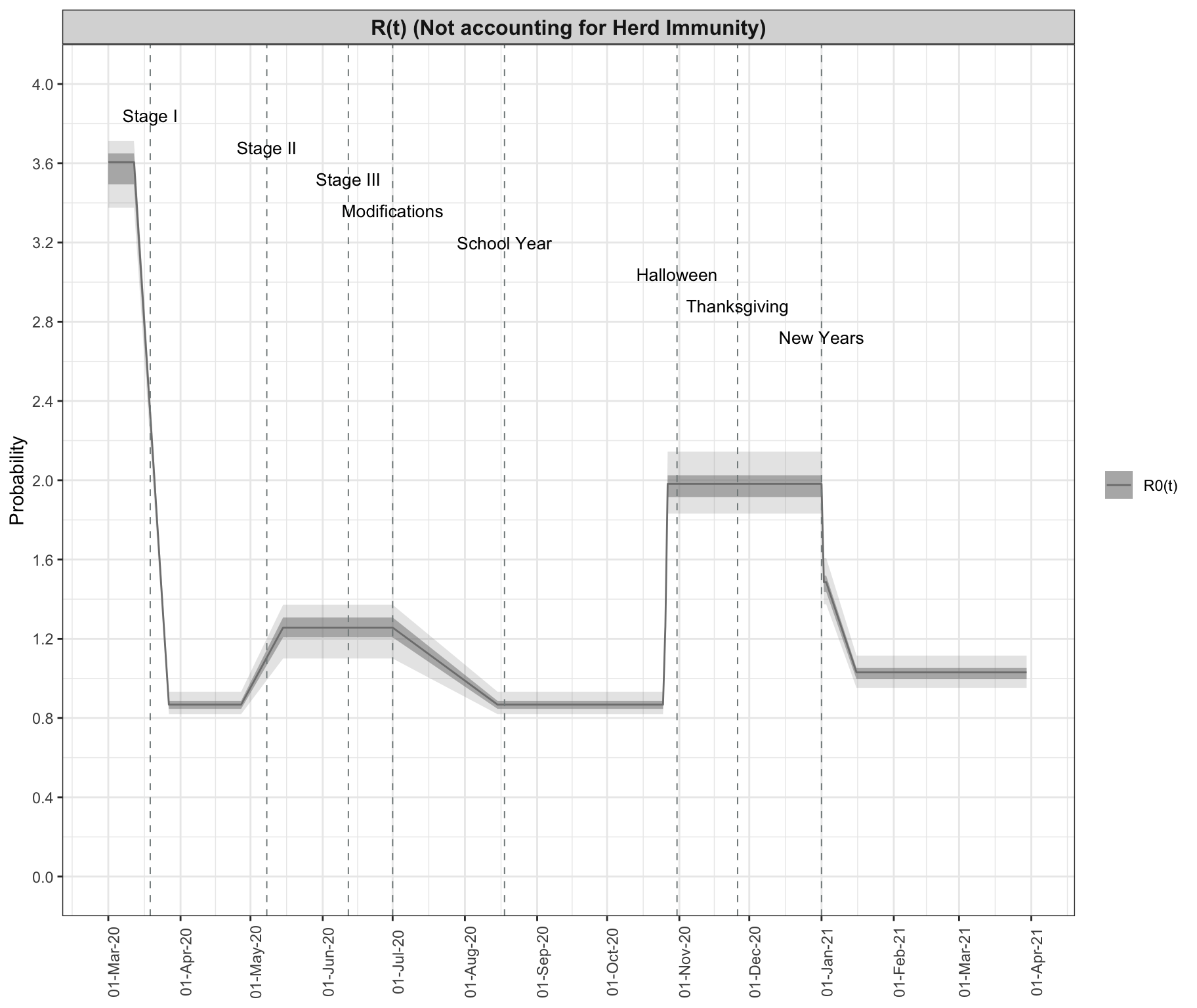### Fraction of Observed Infections, $$r(t)$$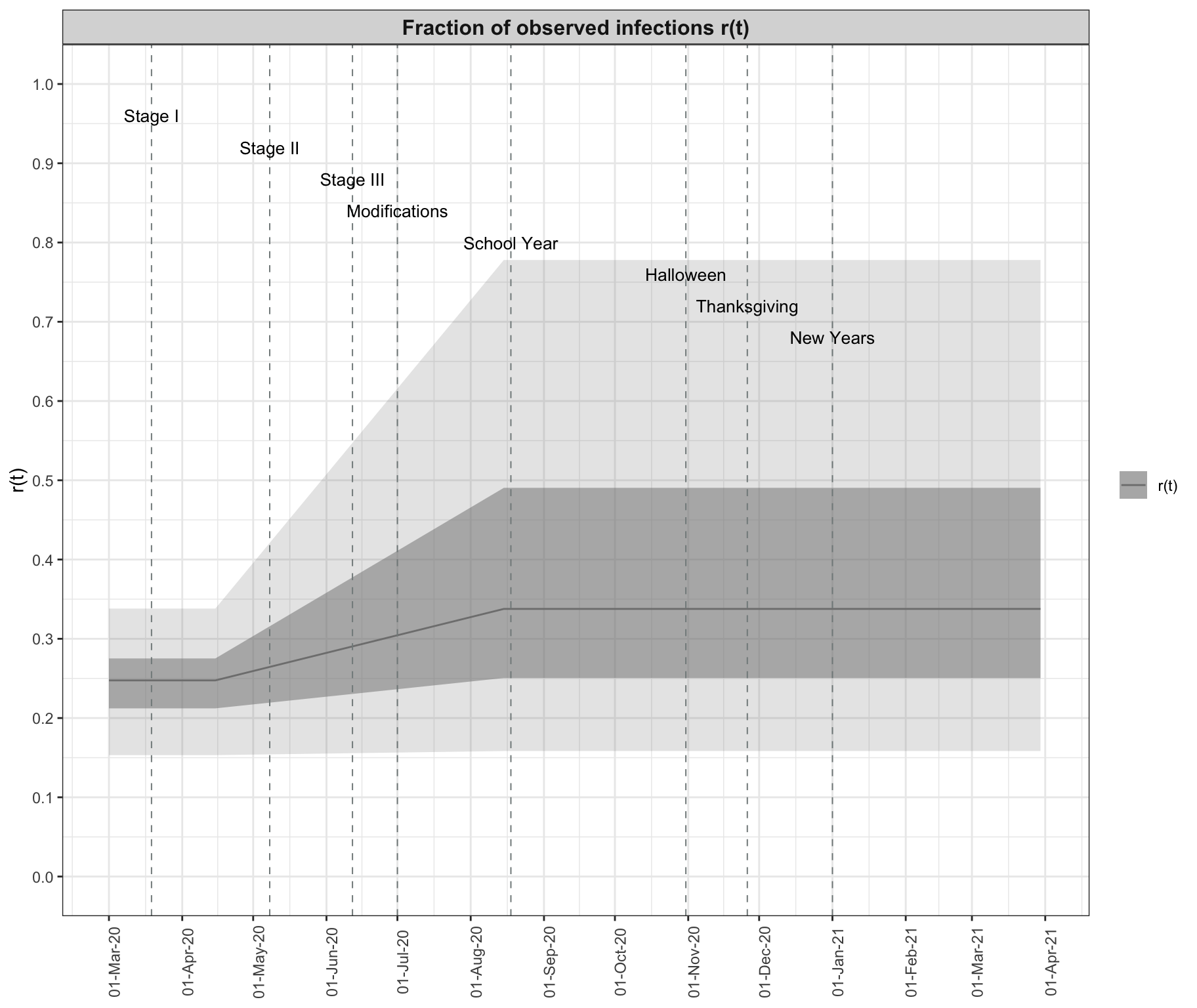### Probabilities of severe illness

• Probability of hospitalization given infection, $$\alpha(t)$$

• Probability of ICU admission given hospitalization, $$\kappa(t)$$

• Probability of death given ICU admission, $$\delta(t)$$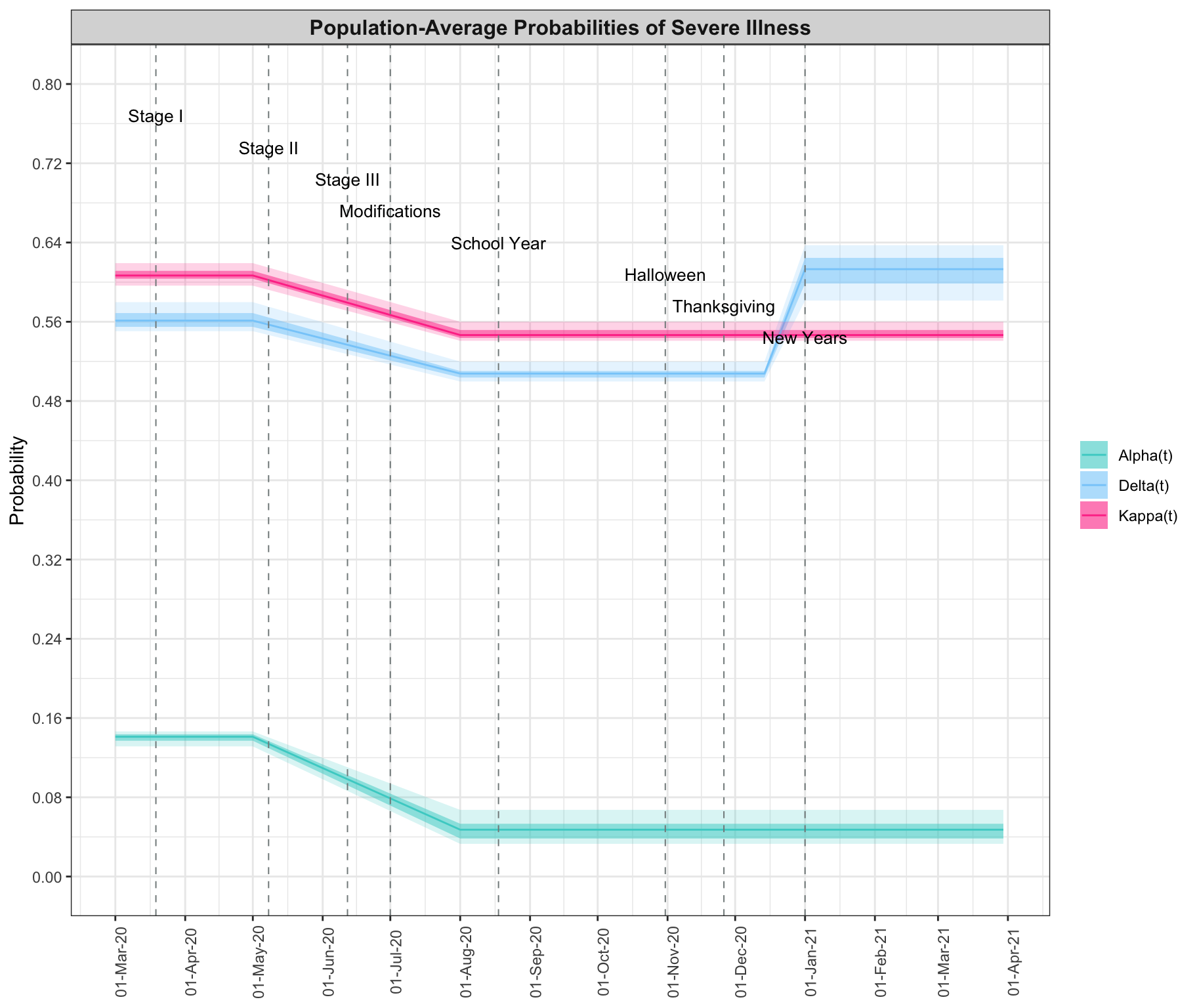{-}

## Tables: Parameter estimates

### $$R0$$, $$r(t)$$, $$\mu(t)$$

mean (95% CI)
R0 3.573 (3.375,3.712)
R(t) 2020-03-27 0.869 (0.82,0.933)
R(t) 2020-05-15 1.251 (1.101,1.372)
R(t) 2020-11-26 1.98 (1.832,2.144)
r(t) 2020-04-15 0.245 (0.153,0.338)
r(t) 2020-08-15 0.385 (0.158,0.778)

### Probabilities of severe illness

• Probability of hospitalization given infection, $$\alpha(t)$$

• Probability of ICU admission given hospitalization, $$\kappa(t)$$

• Probability of death given ICU admission, $$\delta(t)$$

mean (95% CI) Alpha_t mean (95% CI) Kappa_t mean (95% CI) Delta_t
2020-05-01 0.14 (0.131,0.146) 0.607 (0.596,0.619) 0.563 (0.551,0.58)
2020-08-01 0.048 (0.033,0.067) 0.548 (0.541,0.56) 0.508 (0.5,0.52)

### CFR and IFR

CFR mean (95% CI) IFR mean (95% CI)
2020-03-01 0.0081 (0.0012,0.0182) 0.002 (0.0003,0.0048)
2020-03-15 0.008 (0.005,0.0115) 0.002 (0.0009,0.0033)
2020-04-01 0.0146 (0.0122,0.0176) 0.0036 (0.002,0.0052)
2020-04-15 0.0251 (0.022,0.0286) 0.0062 (0.0034,0.009)
2020-05-01 0.0326 (0.0288,0.0368) 0.008 (0.0045,0.0116)
2020-05-15 0.0353 (0.0313,0.0398) 0.0087 (0.0049,0.0126)
2020-06-01 0.0342 (0.0301,0.0396)